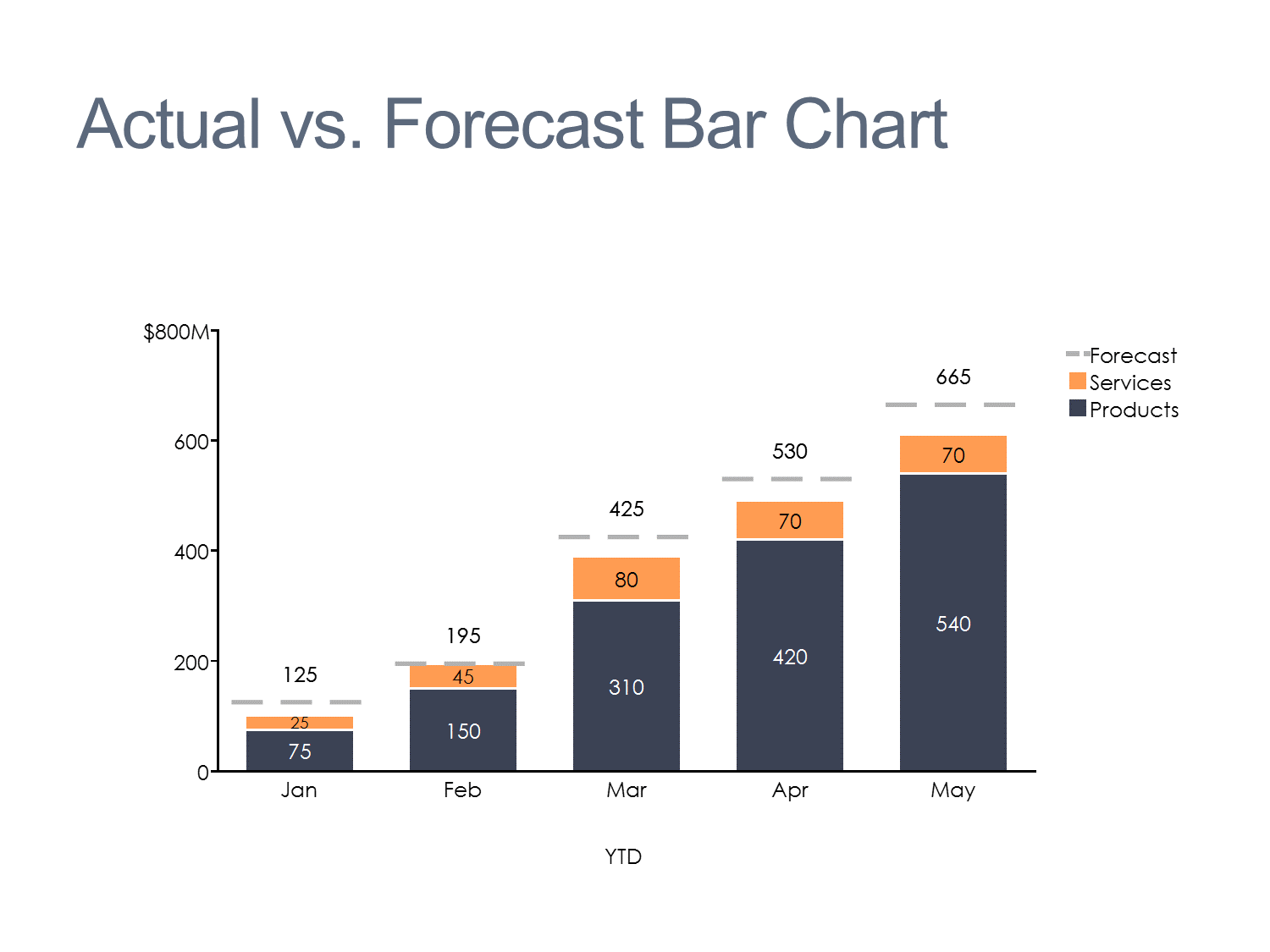# Can I use an area chart to compare YTD actual results to forecast?

This question was submitted to Ask an Expert.

You can use an area chart to show how YTD results (sales \$ or volume, profit, etc) compared to forecast, but only when the actual results are less than forecast.In this case, the forecast series values are the variance between the total of the actual series (products and services) and the forecast. I showed an overall forecast, but you could also show the forecast for each series (products and services). If your actual results exceeded the forecast, however, the forecast values would be negative. The area chart type does not accommodate negative values so I would recommend a different chart type.

I would use a stacked bar chart. I would include a forecast series (actual forecast values, not the variance) as a net line on the chart. This makes it easy to see how the YTD results for each month compares to the YTD forecast. Here's the same data in a stacked bar.